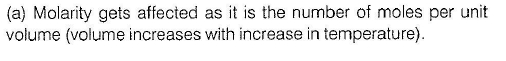Courses

# Retro (Past 13 Years) JEE Main (Liquid Solution)

## 31 Questions MCQ Test Chemistry for JEE Advanced | Retro (Past 13 Years) JEE Main (Liquid Solution)

Description
This mock test of Retro (Past 13 Years) JEE Main (Liquid Solution) for JEE helps you for every JEE entrance exam. This contains 31 Multiple Choice Questions for JEE Retro (Past 13 Years) JEE Main (Liquid Solution) (mcq) to study with solutions a complete question bank. The solved questions answers in this Retro (Past 13 Years) JEE Main (Liquid Solution) quiz give you a good mix of easy questions and tough questions. JEE students definitely take this Retro (Past 13 Years) JEE Main (Liquid Solution) exercise for a better result in the exam. You can find other Retro (Past 13 Years) JEE Main (Liquid Solution) extra questions, long questions & short questions for JEE on EduRev as well by searching above.
QUESTION: 1

### The vapour pressure of acetone at 20°C is 185 torr. When 1.2 g of a non-voiatile substance was dissolved in 100 g of acetone at 20°C, its vapour pressure was 183 torr. The molar mass (g mol-1) of the substance is (JEE Main 2015)

Solution: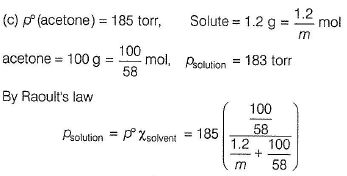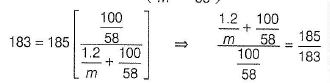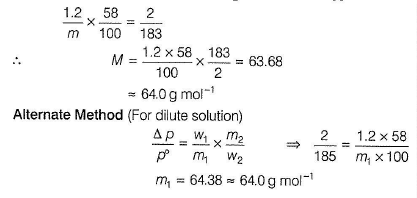QUESTION: 2

### Consider the separate solution of 0.500 M C2H5OH (aq), 0.100 M Mg3(P04)2(aq), 0.250 M KBr (aq) and 0.125 M Na3P04(aq) at 25° C. Which statement is true about these solutions, assuming all salts to be strong electrolytes? (JEE Main 2014)

Solution:

(a)

Salts have been assumed as stronge lectrolytes, van’t Hoff factor,

/ = [1 + (y - 1)x]

y = number of ions from one mole of salt,

x = degree of ionisation = 1

C2H5OH is a non-electrolyte hence, y = 1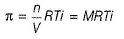Thus, π ∝ Mi.RT being constant. Greater the value of Mi, greater the osmotic pressure.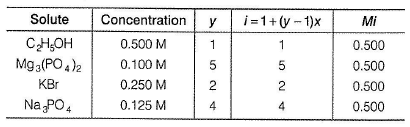Thus, π ∝ Mi, have equal values.

QUESTION: 3

### Kf for water is 1.86 K kg mol-1. If your automobile radiator holds 1.0 kg of water, then how many grams of ethylene glycol (C2H602) must you add to get the freezing point of the solution lowered to -2.8oc ? (AIEEE 2012)

Solution: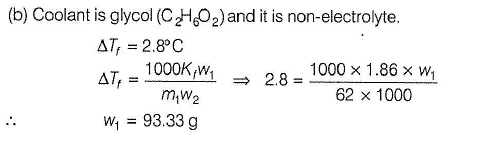QUESTION: 4

A 5.2 molal aqueous solution of methyl alcohol, CH3OH, is supplied. What is the mole fraction of methyl alcohol in the solution ?

(AIEEE 2011)

Solution:

5.2 molal aqueous solution of CH3OH suggests that 5.2 moles ofCH3OH are dissolved in 1000 g of water.

n1(CH3OH) = 5.2 mol

n2(H2O) = 1000/18 = 55.56 mol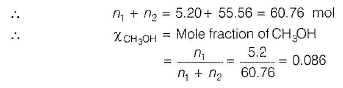QUESTION: 5

Ethylene glycol is used as an a n tifre e z e in a cold climate. Mass of ethylene glycol which should be added to 4 kg of water to prevent it from freezing at - 6" C will be (Kf  for water = 1.86 K kg mol-1 and molar mass of ethylene glycol = 62 g mol-1 )

(AIEEE 2011)

Solution:

ΔTf = Freezing point of H20 - freezing point of ethylene giycol solution

= 0 - (-6°) = 6°
Kf = 1.86° K kg mol-1

w1 = Mass of ethyiene glycol in grams

w2 = Mass of solvent ( H2O) in grams = 4000 g

m1 = Moiar mass of ethylene glycol = 62 g mol-1'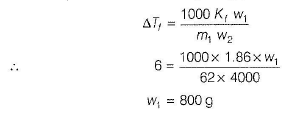QUESTION: 6

The degree of dissociation (α) of a weak electrolyte, AxBy is related to van't Hoff factor (i) by the expression

(AIEEE 2011)

Solution: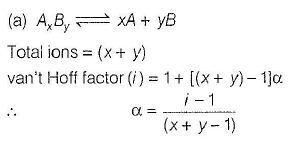QUESTION: 7

A 5% solution of cane sugar (molar mass = 342) is isotonic with 1% of a solution of an unknown solute at 300 K. The molar mass of unknown solute in g/mol is

(AIEEE 2011)

Solution:

(c)

Two or more solutions are said to be isotonic if they have same osmotic pressure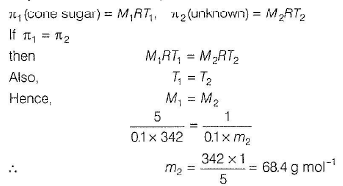QUESTION: 8

The molality of a urea solution in which 0.0100 g of urea, [(NH2)2CO] is added to 0.3000 dm3 of water at STP is

(AIEEE 2011)

Solution: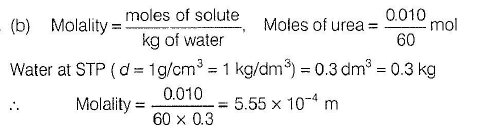QUESTION: 9

If sodium sulphate is considered to be completely dissociated into cations and anions in aqueous solution, the change in freezing point of water (ΔTf), when 0.01 mole of sodium sulphate is dissolved in 1 kg of water, is

(AIEEE 2010)

Solution: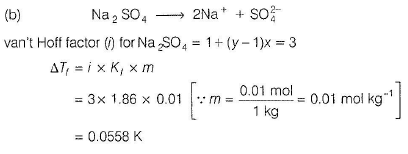QUESTION: 10

On mixing, heptane and octane form an ideal solution. At 373 K, the vapour pressures of the two liquid components (heptane and octane) are 105 kPa and 45 kPa respectively. Vapour pressure of the solution obtained by mixing 25 g of heptane and 35 g of octane will be (molar mass of heptane = 100 g mol-1 and of octane= 114 g mol-1).

(AIEEE2010)

Solution: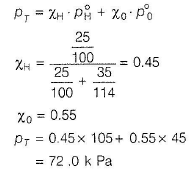QUESTION: 11

Two liquids X and Yform an ideal solution at 300 K, vapour pressure of the solution containing 1 mole of X and 3 moles of Y is 550 mm Hg. At the same temperature, if 1 mole of Y is further added to this solution, vapour pressure of the solution increases by 10 mm Hg. Vapour pressure (in mm Hg) of X and Y in their pure states will be, respectively

(AIEEE2009)

Solution: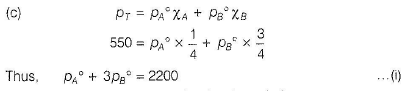When 1 mole of Y is further added to the solution, vapour pressure of a solution becomes 560 mm Hg.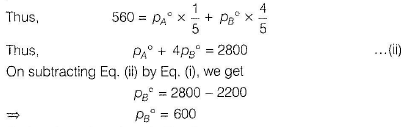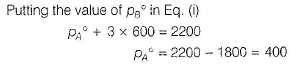QUESTION: 12

A binary liquid solution is prepared by mixing n-heptane and ethanol. Which one of the following statements is correct regarding the behaviour of the solution?

(AIEEE 2009)

Solution:

(b)

n-heptane and ethanol forms non-ideal solution. As a result, n-heptane-ethanol molecular interaction is very poor in comparison to ethanol-ethanoi or n-heptane so the resulting gives positive deviation from Raoult’s law.

QUESTION: 13

At 80°C, the vapour pressure of pure liquid 'A' is 520 mm Hg ana tnai of pure liquid ‘B ’ is 1000 mm Hg. If a mixture solution of A' and ‘6 ’ boils at 80°C and 1 atm pressure, the amount of A ' in the mixture is

(1 atm = 760 mm Hg)

(AIEEE 2008)

Solution:

PT = PoXA + PoXB
760 = 520XA+ PoB(1-XA)
⇒ = 0.5
Thus, mole% of A = 50%

PT = PoXA + PoXB
760 = 520XA+ PoB(1-XA)
⇒ = 0.5
Thus, mole% of A = 50%

QUESTION: 14

The vapour pressure of water at 20°C is 17.5 mm Hg. If 18 g of glucose (C6H1206) is added to 178.2 g of water at 20°C, the vapour pressure of the resulting solution will be

(AIEEE 2008)

Solution:

(d)

This problem is related to the relative lowering of vapour pressure as glucose is a non-volatile solute. Thus, according to Raoult’s law,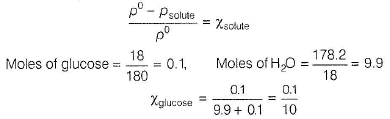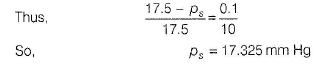QUESTION: 15

A mixture of ethyl alcohol and propyl alcohol has a vapour pressure of 290 mm at 300 K. The vapour pressure of propyl alcohol is 200 mm. If the mole fraction of ethyl alcohol is 0.6, its vapour pressure (in mm) at the same temperature will be

(AIEEE 2007)

Solution:

(a)

According to Raoult’s law,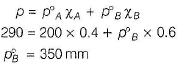QUESTION: 16

The density (in g mL-1)of a 3.60 M sulphuric acid solution that is 29% H2S04 (molar mass = 98 g mol-1) by mass will be

Solution: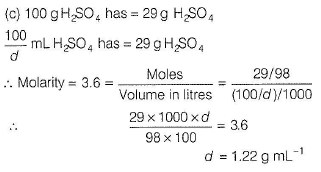QUESTION: 17

A 5.25% solution of a substance is isotonic with a 1.5% solution of urea (molar mass= 60 g mol-1) in the same solvent. If the densities of both the solutions are assumed to be equal to 1.0 g cm-3, molar mass of the substance will be

(AIEEE 2007)

Solution:

(d) O sm otic pressure of isotonic solutions are equal.
Therefore,

π1 = π2

⇒ C1RT = C2RT

⇒ C1 = C2

If densities of both the solutions are equal, then 5.25 g of a substance and 1.5 g of urea are dissolved in the same volume of the solvent, thus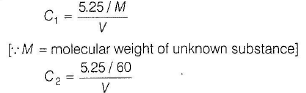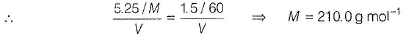QUESTION: 18

Density of a 2.05 M solution of acetic acid in water is 1.02 g / mL. The molality of the solution is

(AIEEE 2006)

Solution: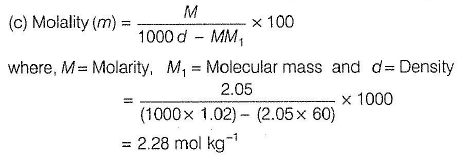QUESTION: 19

18 g of glucose (C6H12O6)is added to 178.2 g of water. The vapour pressure of water for this aqueous solution at 100°C is

(AIEEE 2006)

Solution:

(d) According to Raoult’s law, relative lowering of vapour pressure,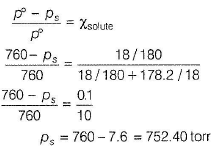QUESTION: 20

Two solutions of a substance (non-electrolyte) are mixed in the following manner. 480 mL of 1.5 M first solution + 520 mL of 1.2 M second solution. What is the molarity of the final mixture?

(AIEEE 2005)

Solution:

(b) The molarity of the resulting solution is given by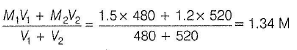QUESTION: 21

Equimolar solutions in the same solvent have

(AIEEE 2005)

Solution:

(b) Boiling point and freezing point depend on Kb (molal elevation constant) and Kf (molal depression constant) of the solvent. Thus, equimolar solution (of the non-electrolyte), will have same boiling point and also same freezing point.

ΔTf = Kf x molality

ΔTb = Kb x molality

Note In this question, nature of solu te has not been mentioned. Hence, vre have assumed that solute is non-electrolyte.

QUESTION: 22

Benzene and toluene form nearly ideal solutions. At 20°C, the vapour pressure of benzene is 75 torr and that of toluene is 22 torr. The partial vapour pressure of benzene at 20°C for a solution containing 78 g of benzene and 46 g of toluene in torr is

(AIEEE 2005)

Solution:

(d) Mixture contains 78 g benzene = 1 mol benzene and

46 g toluene = 0.5mol toluene

Total moles of benzene and toluene = 1.5 mol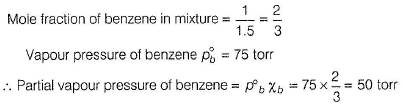QUESTION: 23

If α is the degree of dissociation of Na2SO4, the van’t Hoff factor (/) used for calculating the molecular mass is

(AIEEE 2005)

Solution: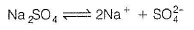van't Hoff factor, i = [1+ (y - 1) α]

where y is the number of ions from one mole solute,

(in this case = 3), α is the degree of dissociation, i = (1+ 2 α)

QUESTION: 24

6.02 x 102° molecules of urea are present in 100 mL of its solution. The concentration of urea solution is

(Avogadro constant, NA = 6.02 x 1023 mol-1)

(AIEEE 2004)

Solution:

(b) Avogadro’s number, NA = 6.02 x 1023 molecules = 1 mol

6,02x 1020 molecules = 0.001 mol in 100 mL or (0.1 L) solution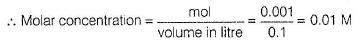QUESTION: 25

To neutralise completely 20 mL of 0.1 M aqueous solution of phosphorous acid (H3P03), the volume of 0.1 M aqueous KOH solution required is

(AIEEE 2004)

Solution:

(c)

H3PO3 is a dibasic acid (i.e. contains two ionisable protons attached directly to O).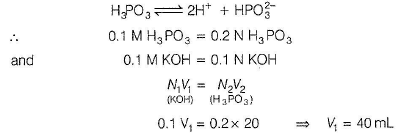QUESTION: 26

Which of the following liquid pairs shows a positive deviation from Raoult’s law?

(AIEEE 2004)

Solution:

(b) Water and hydrochloric acid; and water and nitric acid form miscible solutions. They show negative deviation.
In case of CH3COCH3 and CHCI3, there is interaction between them, thus force of attraction between CH3COCH3....CHCI3 is larger than between CHCI3....CHCI3 or CH3COCH,....CH3COCH3 and thus, vapour pressure is less than expected—a negative deviation.

In case ofCH3OH , there is association by intermolecular H-bonding. When benzene is added toCH3OH, H-bonding breaks and thus, force of attraction between CH3OH and benzene molecule is smaller than between CH3OH or benzene molecules (in pure state). Vapour pressure of mixture is greater than expected—a positive deviation.QUESTION: 27

Which one of the following aqueous solutions will exhibit highest boiling point?

(AIEEE 2004)

Solution:

(a) The colligative property will be given as ΔTb = i x  Kx m

Where, ΔTb= Elevation in boiling point

i = van’t Hoff factor

Kb = molal elevation constant

m = molality of the solution

From the above equation, it is clear that,

ΔTb ∝ i

This suggests that higher the value of i, larger is the boiling point of the solution.
For 100% dissociation, i = number of ions produced by one mole of solute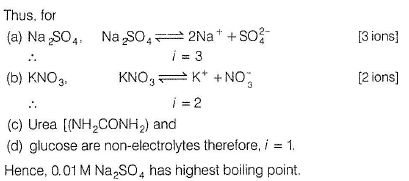QUESTION: 28

Which one of the following statements is false?

(AIEEE 2004)

Solution: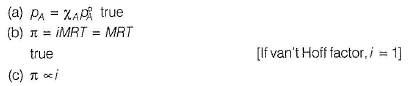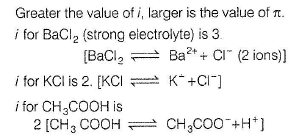But CH3COOH is weak electrolyte than KCI.

i for sucrose is 1 as it is a non-electrocyte.
Thus, i for BaCI2> KCI > CH3COOH > sucrose Thus, (c) is also true.

(d) ΔTf = Kfm.
Kf is dependent on solvent.
Thus, freezing points = [T (solvent) - ATf] are different.
Thus, (d) is false.

QUESTION: 29

If liquid A and B form an ideal solution, the

(AIEEE 2003)

Solution: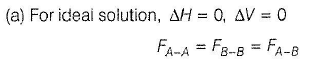QUESTION: 30

In a 0.2 molal aqueous solution of a weak acid HX, the degree of ionisation is 0.3. Taking Kf for water as 1.85, the freezing point of the solution will be nearest to

(AIEEE 2003)

Solution: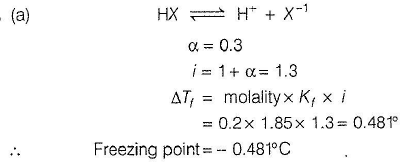QUESTION: 31

Which of the following concentration factor is affected by change in temperature?

(AIEEE 2002)

Solution: